+
Introduction to Inequalities
Solving Linear Equations and Inequalities
0
of 0 possible points

# Introduction to Inequalities

Author: Sophia Tutorial
##### Description:

Identify the correct compound inequality for a given number line.

(more)

Sophia’s self-paced online courses are a great way to save time and money as you earn credits eligible for transfer to many different colleges and universities.*

No credit card required

37 Sophia partners guarantee credit transfer.

299 Institutions have accepted or given pre-approval for credit transfer.

* The American Council on Education's College Credit Recommendation Service (ACE Credit®) has evaluated and recommended college credit for 33 of Sophia’s online courses. Many different colleges and universities consider ACE CREDIT recommendations in determining the applicability to their course and degree programs.

Tutorial
what's covered
1. What is an inequality?
2. Plotting inequalities on a number line
1. "Less Than" Inequalities
2. "Greater Than" Inequalities
3. Compound Inequalities

# 1. What is an inequality?

When we have an equation such as x = 4 we have a specific value for our variable. With inequalities we will give a range of values for our variable. To do this we will not use equals, but one of the following symbols: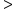Greater than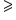Greater than or equal to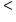Less than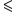Less than or equal to

The above symbols are inequality symbols. They relate two quantities as not being equal to each other. Let's look at a definition of an inequality:

If we have an expression such as x < 4, this means our variable can be any number smaller than 4 such as − 2, 0, 3, 3.9 or even 3.999999999 as long as it is smaller than 4. If we have an expression such as x greater or equal than − 2, this means our variable can be any number greater than or equal to −2, such as 5, 0, −1, −1.9999, or even −2.

term to know
Inequality
a mathematical statement that two quantities are not equal in value.

# 2. Plotting inequalities on a number line

Because we don’t have one set value for our variable, it is often useful to draw a picture of the solutions to the inequality on a number line. We will start from the value in the problem and bold the lower part of the number line if the variable is smaller than the number, and bold the upper part of the number line if the variable is larger. The value itself we will mark with brackets, eitherorfor "less than" or "greater than" respectively, andorfor "less than or equal to" or "greater than or equal to" respectively.

Once the graph is drawn we can quickly convert the graph into what is called interval notation. Interval notation gives two numbers, the first is the smallest value, the second is the largest value. If there is no largest value, we can use ∞ (infinity). If there is no smallest value, we can use − ∞ negative infinity. If we use either positive or negative infinity we will always use a curved bracket for that value.

2a. "Less Than" Inequalities

Graph the inequality, x < 2, and give the interval notation.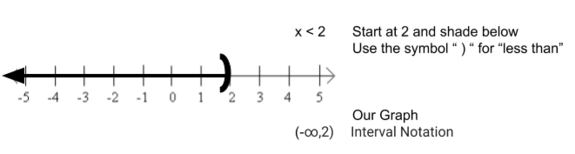For the "less than" inequality, x < 2, we know that x cannot be exactly equal to two but can be anything smaller. First, we show that x cannot be exactly equal to two by putting aor an open circle on the number line right at the two. An open circle means that the value for x cannot be exactly equal to the value that it's on top of. We also want to show that x can be less than two. We can use an arrow pointing to the left, or pointing to the numbers that are less than two. The region above represents all the solutions to the inequality, x is less than 2, and any value in the highlighted region is going to satisfy this inequality.

Keep in mind that if this inequality was x ≤ 2, then we would have used a bracketor a closed circle.

2b. "Greater Than" Inequalities

Graph the inequality, y ≥ -1, and give the interval notation.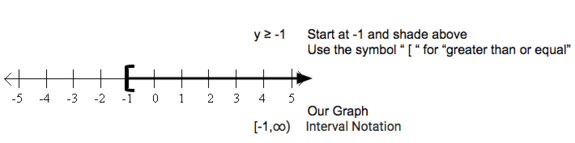For the "greater than or equal to" inequality, y ≥ -1, we need to show that y can be exactly equal to negative one. We can do that putting aor a closed circle on the number line right at negative one. Next, we want to show that y can also be anything greater than negative one. We can use an arrow pointing to the right, towards the numbers that are greater than negative one. This represents all the solutions to the inequality, y ≥ -1. Any value of y in this highlighted region, including negative one, is going to satisfy the inequality.

2c. Compound Inequalities

Graph the inequality,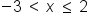, and give the internal notation.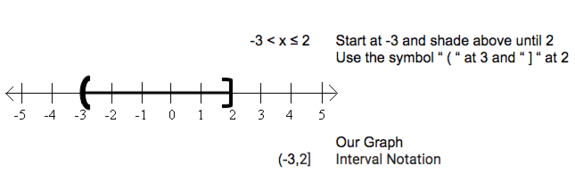For the compound inequality,, the values for x that are going to satisfy this inequality have to be bigger than negative three and less than or equal to two. We start by putting a ""or an open circle at negative three because we see that x cannot be exactly equal to negative three. Then we are going to use the "" or closed circle at two because we know that x can be equal exactly to two.

We want to show that x is greater than negative three but that x is also smaller than or equal to two. We can see that our solution is going to be in between these two points. Using this highlighted region, we can see that any value that's in this region is going to satisfy the inequality. Any number greater than negative three or less than or equal to two will satisfy this inequality.

summary
When thinking about what is an inequality, we use inequalities to show that two quantities are not equal in value. We can use a number line to show a range of values that can satisfy an inequality expression. When we're plotting an inequality on a number line, we use an open circle or parentheses for the symbols "less-than" and "greater-than" and use a filled in circle or brackets for the symbols "less-than or equal to" and "greater-than or equal to."

Source: Adapted from "Beginning and Intermediate Algebra" by Tyler Wallace, an open source textbook available at: http://wallace.ccfaculty.org/book/book.html

Terms to Know
Inequality

a mathematical statement that two quantities are not equal in value

Rating